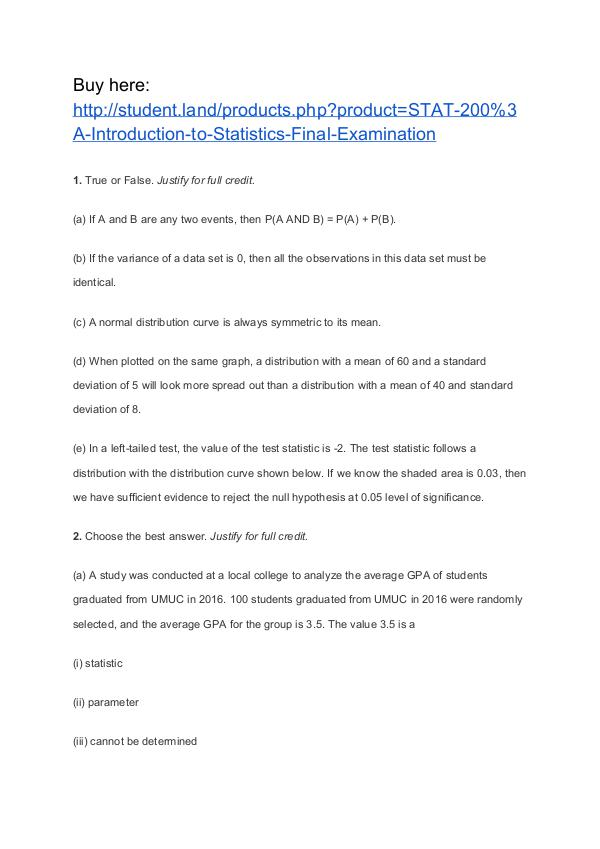Cliquez pour lire
• Commentaires

# STAT 200: Introduction to Statistics Final ExaminationSTAT 200

Publié sur dans “Astuces”, langue – English. 9 pages.
1. True or False. Justify for full credit. (a) If A and B are any two events, then P(A AND B) = P(A) + P(B). (b) If the variance of a data set is 0, then all the observations in this data set must be identical. (c) A normal distribution curve is always symmetric to its mean. (d) When plotted on the same graph, a distribution with a mean of 60 and a standard deviation of 5 will look more spread out than a distribution with a mean of 40 and standard deviation of 8. Plus
1. True or False. Justify for full credit. (a) If A and B are any two events, then P(A AND B) = P(A) + P(B). (b) If the variance of a data set is 0, then all the observations in this data set must be identical. (c) A normal distribution curve is always symmetric to its mean. (d) When plotted on the same graph, a distribution with a mean of 60 and a standard deviation of 5 will look more spread out than a distribution with a mean of 40 and standard deviation of 8. Plus
S'abonner GRATUITEMENT